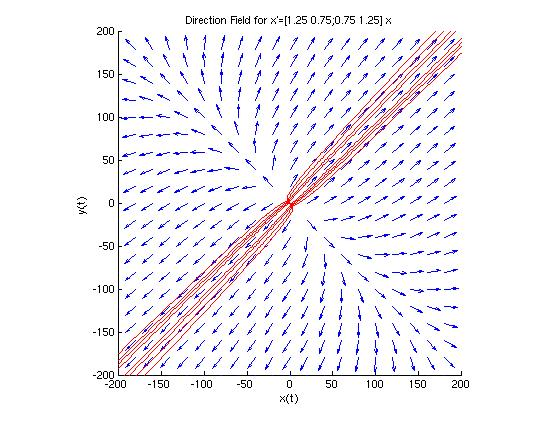## Problem #6

Find the general solution of the given system of equations and describe the behavior of the solution as t approaches infinity. Also draw a direction field and plot a few trajectories for the system.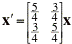To solve this differential equation, we first need to find the eigenvalues and eigenvectors of the matrix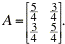In MATLAB, this is a simple one-line command, once the matrix has been entered:

```  >> A=[5/4 3/4;3/4 5/4];
>> [V,D]=eig(A)
V =
-0.7071    0.7071
0.7071    0.7071
D =
0.5000         0
0    2.0000
>>
```

The matrices V and D are the matrices that diagonalize the matrix A. That is, the columns of V are the eigenvectors of A that correspond to the eigenvalues of A found in the corresponding column of the diagonal matrix D. Recall that we can scale the eigenvectors by any value we wish. Thus, for the solution below, I chose to scale the eigenvectors to [-1;1] and [1;1] to make the formula prettier (but no different). Using formula (25) in section 7.5 of the text, we find the general solution of the given differential equation is: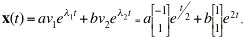or equivalently, as parametric functions,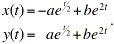The MATLAB module dirfield2d.m will plot the direction field of a 2x2 linear system x' = A x. It also provides the option of plotting individual trajectories on the direction field, when given an user-specified initial point. Here we will run 'dirfield2d' for the matrix A.

```  >> dirfield2d
Enter the 2x2 matrix A as [a b;c d] => [5/4 3/4;3/4 5/4]

Enter the window dimensions for the direction field:
Enter the smallest x value => -5
Enter the largest  x value => 5
Enter the smallest y value => -5
Enter the largest  y value => 5
Warning: Divide by zero.
> In dirfield2d at 87
Warning: Divide by zero.
> In dirfield2d at 88

Would you like to plot a solution curve on this direction field? (y or n) => n
>>
```

Note: The 'divide by zero' warnings are true, but we can ignore them. We'll get that warning anywhere the tangent line becomes vertical. It is not a problem.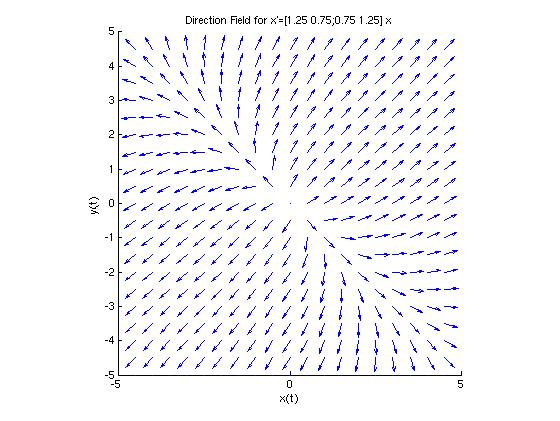If we answer 'y' instead of 'n' to the last question in dirfield2d, we are prompted to enter the coordinates of an initial condition that determines the integral curve (trajectory) on the direction field that corresponds to the particular solution to the initial value problem.

```  Would you like to plot a solution curve on this direction field? (y or n) => y
Enter x(0) for this initial value problem => -3
Enter y(0) for this initial value problem => 4

Would you like to plot another solution curve (y or n) => y
Enter x(0) for this initial value problem => -2
Enter y(0) for this initial value problem => 3

Would you like to plot another solution curve (y or n) => n
>>
```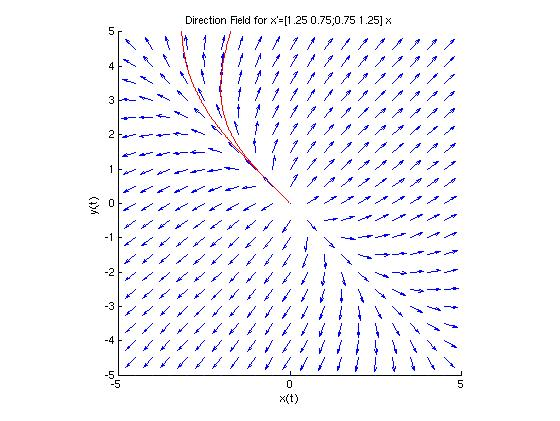Here is the phase plot that arises when we plot the 16 trajectories with initial conditions (x(0), y(0)) as shown below:

 (-3, 4), (3, -4), (-10, 0), (10, 0), (-2, 3), (2, -3), (-6, 0), (6, 0), (-1, 2), (1, -2), (-4, 0), (4, 0), (-.5, 1), (.5, -1), (-2, 0), (2, 0)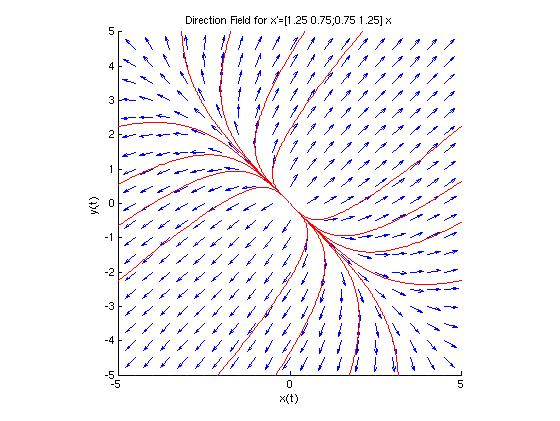If we had chosen an initial condition lying on either of the lines through the eigenvectors, then the solution is the line through that eigenvector. In the phase plot below, these two solutions are plotted in aqua.

```  >> fplot('x',[xmin,xmax],'c--');    % Plot y = x with dashed cyan line
>> fplot('-x',[xmin,xmax],'c--');   % Plot y = -x with dashed cyan line
>>
```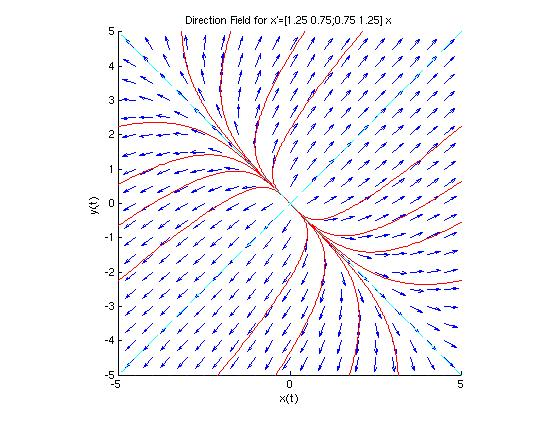When analyzing the phase plot for this system, we need to pay attention to two things involving the eigenvalues and eigenvectors of the matrix A. The eigenvalues and eigenvectors of A are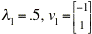and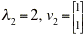.

First, the origin is an unstable node for this problem because the trajectories on the phase plot are all pulled away from the origin. Because both eigenvalues are positive, the exponential parts of the solution go to infinity so both x(t) and y(t) go to infinity as t approaches infinity. As a result, the trajectories each move away from the origin. We can see that by watching the arrows on the direction field.

Secondly, we see that nearly all trajectories start in the direction of v1, but are eventually pulled toward the line through v2. Here, it's the magnitudes of the eigenvalues that are important. The functions x(t) and y(t) are each dominated by exponential part involving the larger of the positive eigenvalues (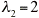), and thus eventually they each behave like the function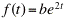. This means the parametric function (x(t), y(t)) eventually approaches the parametric function (f(t), f(t)), which is the line y = x. If we re-ran dirfield2d with a bigger window, we'd see the effect of this more clearly. The direction field below looks the same as the previous ones, but now it's drawn in a 200x200 window (instead of 5x5). The trajectories drawn here are the same 16 trajectories we drew previously. It is now more obvious that they eventually approach the line y = x.